# Vectors and Linear Algebra

Most of the content on this page is adapted from The HyperPhysics Website – Georgia State University, the MIT’s Open Courseware Site, and Wikipedia.

This page is a complete study guide for learning Vectors and Linear Algebra.

# Basic Vector Operations

Both a magnitude and a direction must be specified for a vector quantity, in contrast to a scalar quantity which can be quantified with just a number. Any number of vector quantities of the same type (i.e., same units) can be combined by basic vector operations.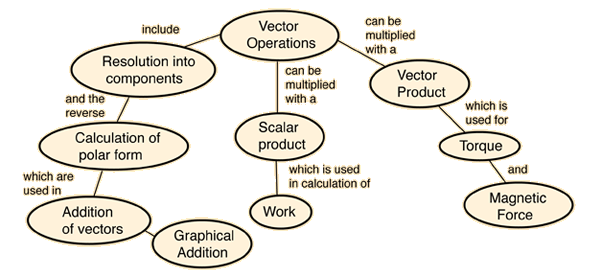Caution! This is a large HTML document. You need to wait for it to load completely in order for all the links above to operate.
Index

Math of vectors

 HyperPhysics***** Mechanics R Nave
Go Back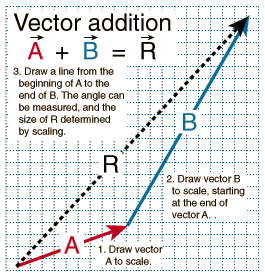Adding two vectors A and B graphically can be visualized like two successive walks, with the vector sum being the vector distance from the beginning to the end point. Representing the vectors by arrows drawn to scale, the beginning of vector B is placed at the end of vector A. The vector sum R can be drawn as the vector from the beginning to the end point. The process can be done mathematically by finding the components of A and B, combining to form the components of R, and then converting to polar form.
Index

Vector concepts

 HyperPhysics***** Mechanics R Nave
Go Back

# Example of Vector Components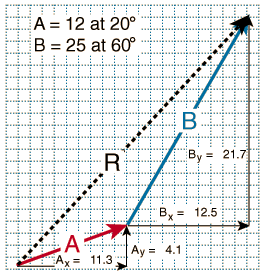Finding the components of vectors for vector addition involves forming a right triangle from each vector and using the standard triangle trigonometry.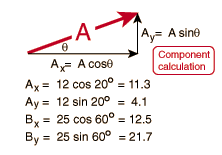The vector sum can be found by combining these components and converting to polar form.
Index

Vector concepts

 HyperPhysics***** Mechanics R Nave
Go Back

# Polar Form Example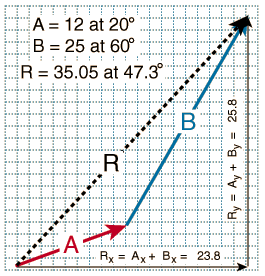After finding the components for the vectors A and B, and combining them to find the components of the resultant vector R, the result can be put in polar form by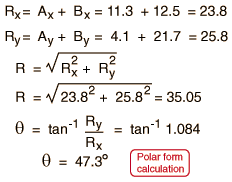Some caution should be exercised in evaluating the angle with a calculator because of ambiguities in the arctangent on calculators.
Index

Vector concepts

 HyperPhysics***** Mechanics R Nave
Go Back

# Combining Vector Components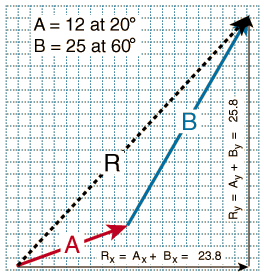After finding the components for the vectors A and B, these components may be just simply added to find the components of the resultant vector R.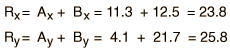The components fully specify the resultant of the vector addition, but it is often desirable to put the resultant in polar form.
Index

Vector concepts

 HyperPhysics***** Mechanics R Nave
Go Back

# Resolving a Vector Into Components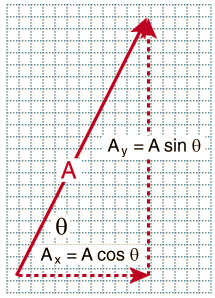Vectors are resolved into components by use of the triangle trig relationships. You may change the length or angle of the polar form of the vector, and the components will be calculated below. For vector A= at angle  degrees,the horizontal component is =  and the vertical component is =   The input to the boxes for units is arbitrary; they serve to emphasize that the process of vector addition is independent of the units of the vector.

Note: this Javascript routine does not work for angle exactly equal to 90° .

Index

Vector concepts

 HyperPhysics***** Mechanics R Nave
Go Back

# Magnitude and Direction from Components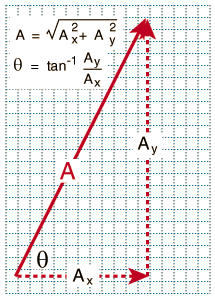If the components of a vector are known, then its magnitude and direction can be calculated with the use of the Pythagorean relationship and triangle trig. This is called the polar form of the vector. If the horizontal component is = and the vertical component is =  ,then the magnitude is =  and the angle is =  degrees. The input to the boxes for units is arbitrary; they serve to emphasize that the process of vector addition is independent of the units of the vector. Some caution should be exercised in evaluating the angle with a calculator because of ambiguities in the arctangent on calculators.
Index

Vector concepts

 HyperPhysics***** Mechanics R Nave
Go Back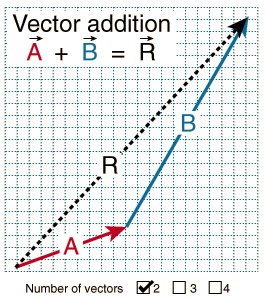Vector addition involves finding vector components, adding them and finding the polar form of the resultant. The addition of vector A= at  degrees, and vector B= at  degrees,yields components:+  =+  =  The resultant has magnitude R =  and angle =  degrees.
Index

Vector concepts

 HyperPhysics***** Mechanics R Nave
Go Back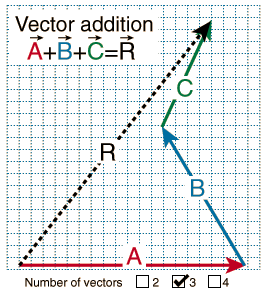Vector addition involves finding vector components, adding them and finding the polar form of the resultant. The addition of vectors A= at  degrees, B= at  degrees,and C= at  degreesyields components: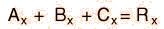+  +  =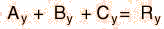+  +  =  The resultant has magnitude R =  and angle =  degrees.
Index

Vector concepts

 HyperPhysics***** Mechanics R Nave
Go Back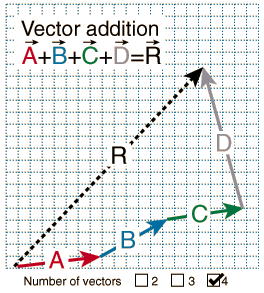Vector addition involves finding vector components, adding them and finding the polar form of the resultant. The addition of vectors A= at  degrees, B= at  degrees, C= at  degrees, D= at  degrees,yields components: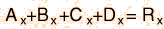+++=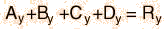+++= The resultant has magnitude R =  and angle =  degrees.
Index

Vector concepts

 HyperPhysics***** Mechanics R Nave
Go Back

# Coordinate Systems

## Cartesian coordinate system

The prototypical example of a coordinate system is the Cartesian coordinate system. In the plane, two perpendicular lines are chosen and the coordinates of a point are taken to be the signed distances to the lines.

In three dimensions, three perpendicular planes are chosen and the three coordinates of a point are the signed distances to each of the planes. This can be generalized to create n coordinates for any point in n-dimensional Euclidean space.

# Vectors as Matrices

It is sometimes useful for systems of equations and groups of vectors to be arranged in the form of a matrix.

### Vector Products

It is also sometimes useful to use a Matrix when dealing with Vector Multiplication.

### Matrix notation

The definition of the cross product can also be represented by the determinant of a formal matrix:$\mathbf{a}\times\mathbf{b}= \begin{vmatrix} \mathbf{i} & \mathbf{j} & \mathbf{k} \\ a_1 & a_2 & a_3 \\ b_1 & b_2 & b_3 \\ \end{vmatrix}.$

This determinant can be computed using Sarrus’ rule or Cofactor expansion.

Using Sarrus’ Rule, it expands to$\mathbf{a}\times\mathbf{b}= \mathbf{i}a_2b_3 + \mathbf{j}a_3b_1 + \mathbf{k}a_1b_2 - \mathbf{i}a_3b_2 - \mathbf{j}a_1b_3 - \mathbf{k}a_2b_1.$

Using Cofactor expansion along the first row instead, it expands to$\mathbf{a}\times\mathbf{b}= \begin{vmatrix} a_2 & a_3\\ b_2 & b_3 \end{vmatrix} \mathbf{i} - \begin{vmatrix} a_1 & a_3\\ b_1 & b_3 \end{vmatrix} \mathbf{j}+ \begin{vmatrix} a_1 & a_2\\ b_1 & b_2 \end{vmatrix} \mathbf{k}$

which gives the components of the resulting vector directly.

### Geometric meaningFigure 2: The volume of a parallelepiped using dot and cross-products; dashed lines show the projections of c onto a × b and of a onto b × c, a first step in finding dot-products.

The magnitude of the cross product can be interpreted as the positive area of the parallelogram having a and b as sides (see Figure 1):$A = | \mathbf{a} \times \mathbf{b}| = | \mathbf{a} | | \mathbf{b}| \sin \theta. \,\!$

Indeed, one can also compute the volume V of a parallelepiped having ab and c as sides by using a combination of a cross product and a dot product, called scalar triple product (see Figure 2):$V = |\mathbf{a} \cdot (\mathbf{b} \times \mathbf{c})|.$

Figure 2 demonstrates that this volume can be found in two ways, showing geometrically that the identity holds that a “dot” and a “cross” can be interchanged without changing the result. That is:$V =\mathbf{(a \times b) \cdot c} = \mathbf{a \cdot (b \times c)} \ .$

Because the magnitude of the cross product goes by the sine of the angle between its arguments, the cross product can be thought of as a measure of “perpendicularness” in the same way that the dot product is a measure of “parallelness”. Given two unit vectors, their cross product has a magnitude of 1 if the two are perpendicular and a magnitude of zero if the two are parallel.

## Determinants

In algebra, the determinant is a special number associated with any square matrix. The fundamental geometric meaning of a determinant is a scale factor or coefficient for measure when the matrix is regarded as a linear transformation. Thus a 2 × 2 matrix with determinant 2 when applied to a set of points with finite area will transform those points into a set with twice the area. Determinants are important both in calculus, where they enter the substitution rule for several variables, and in multilinear algebra.

When its scalars are taken from a field F, a matrix is invertible if and only if its determinant is nonzero; more generally, when the scalars are taken from a commutative ring R, the matrix is invertible if and only if its determinant is a unit of R. Determinants are not that well-behaved for noncommutative rings.

The determinant of a matrix A is denoted det(A), or without parentheses: det A. An alternative notation, used for compactness, especially in the case where the matrix entries are written out in full, is to denote the determinant of a matrix by surrounding the matrix entries by vertical bars instead of the usual brackets or parentheses. Thus$\begin{vmatrix} a & b & c\\d & e & f\\g & h & i \end{vmatrix}\$ denotes the determinant of the matrix$\begin{bmatrix}a&b&c\\ d&e&f\\g&h&i\end{bmatrix}.$

For a fixed nonnegative integer n, there is a unique determinant function for the n×n matrices over any commutative ring R. In particular, this unique function exists when R is the field of real or complex numbers.

### Interpretation as the area of a parallelogramThe area of the parallelogram is the absolute value of the determinant of the matrix formed by the vectors representing the parallelogram’s sides.

The 2×2 matrix$A = \begin{bmatrix} a & b\\c & d \end{bmatrix}\,$

has determinant$\det A = ad - bc.\$

If A is a 2×2 matrix, its determinant det A can be viewed as the oriented area of the parallelogram with vertices at (0,0), (a,b), (a + cb + d), and (c,d). The oriented area is the same as the usual area, except that it is negative when the vertices are listed in clockwise order.

Further, the parallelogram itself can be viewed as the unit square transformed by the matrix A. The assumption here is that a linear transformation is applied to row vectors as the vector-matrix product xTAT, where x is a column vector. The parallelogram in the figure is obtained by multiplying matrix A (which stores the co-ordinates of our parallelogram) with each of the row vectors$\begin{bmatrix} 0 & 0 \end{bmatrix}, \begin{bmatrix} 1 & 0 \end{bmatrix}, \begin{bmatrix} 1 & 1 \end{bmatrix}$ and$\begin{bmatrix}0 & 1\end{bmatrix}$ in turn. These row vectors define the vertices of the unit square. With the more common matrix-vector product Ax, the parallelogram has vertices at$\begin{bmatrix} 0 \\ 0 \end{bmatrix}, \begin{bmatrix} a \\ c \end{bmatrix}, \begin{bmatrix} a+b \\ c+d \end{bmatrix}$ and$\begin{bmatrix} b \\ d \end{bmatrix}$ (note that Ax = (xTAT)T ).

Thus when the determinant is equal to one, then the matrix represents an equi-areal mapping.

### 3-by-3 matrices

The determinant of a 3×3 matrix$A=\begin{bmatrix}a&b&c\\ d&e&f\\g&h&i\end{bmatrix}$

is given by$\det A = aei + bfg + cdh - afh - bdi - ceg.\$

The rule of Sarrus is a mnemonic for this formula: the sum of the products of three diagonal north-west to south-east lines of matrix elements, minus the sum of the products of three diagonal south-west to north-east lines of elements when the copies of the first two columns of the matrix are written beside it as below:$\begin{matrix} \color{red}a & \color{red}b & \color{red}c & a & b \\ d & \color{red}e & \color{red}f & \color{red}d & e \\ g & h & \color{red}i & \color{red}g & \color{red}h \end{matrix} \quad - \quad \begin{matrix} a & b & \color{blue}c & \color{blue}a & \color{blue}b \\ d & \color{blue}e & \color{blue}f & \color{blue}d & e \\ \color{blue}g & \color{blue}h & \color{blue}i & g & h \end{matrix}$

This mnemonic does not carry over into higher dimensions.

## Other Properties of Determinants

Switching two rows in a matrix with change the sign (+/-) of the determinant.

Row replacement operations (adding a constant multiple of one row to another) does nothing to the determinant.

Scaling a row (aka multiplying or dividing any row by a scalar) will multiply or divide the determinant by that scalar also.

# Linear Algebra and Matrices

Groups of multiple vectors and systems of polynomial equations can be placed inside a matrix, which can be useful for a variety of operations. A Polynomial is anything of the form: P = a0 + a1x + a2x2 + a3x3 + a4x4 + … where a0-an are constants. An example would be y = x2 + 2x – 4 or s = 3t4 – 7

It’s important to understand that the linear independence of x and x2 in polynomial equations conform to the same matrix mathematics as vectors which contain linearly independent components such as x, y, and z.

Is the system consistent? This is the first question you ask when you look at any matrix, or a system of polynomials or vectors. You may know from algebra that in order to solve M equations from N unknowns you need at least as many equations as unknowns in order to solve it, and even then a solution isn’t always guarunteed. You may have a case where two equations represent the same line, or where two or more vectors are not linearly independent, in other words, there are vectors which can be generated as multiples or combinations of other vectors. Two vectors are linearly dependent if and only if one is a constant multiple of the other. For example a=(2,4) and b=(4,8) are both along the same exact line if you were to draw them out on a graph, they are both linearly dependent. Another example would be s=(2,1,3,5) and t=(4,2,6,10) since t=2s they are constant multiples of the same vector so they are linearly dependent.

Beyond 2 vectors it gets a bit more tricky to tell linear independence of vectors

So if M < N

An [M x N] Matrix is (M Rows by N Columns):Here is a 4×3 Matrix (4-by-3) for example: